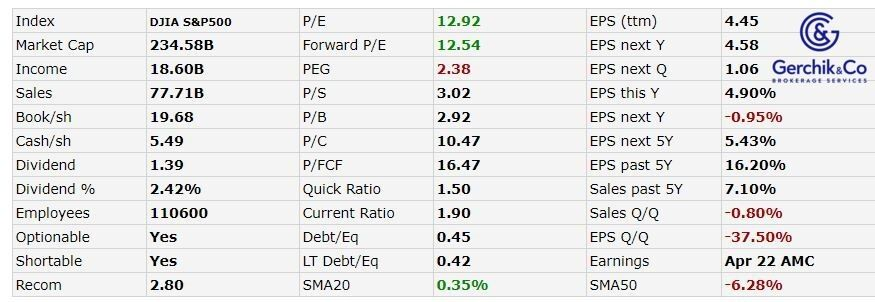# How to make money in stocks. Lesson 33. General conclusion. IntelIn our previous lessons, we explored the key multiples that are used when picking or analyzing stocks. It stands to mention that this information may suffice for individual investors although there is always room to learn. However, this type of assessment is more of a rough analysis and generally lacks thoroughness.

As far as investment funds, banks, and investment companies are concerned, you will have to research them in a more in-depth way using financial modeling and possible algorithms. This is a much more expensive and time-consuming process.

Now let's try to analyze the multiples we have considered previously and which you will definitely come across on exchange websites. This time our focus is on Intel company.

## Intel #INTC

Market Capitalization = Total Number of Shares Allotted by the Company * Current Market Price of each Share = 4.06 billion * \$57.48 = \$233.37 billion

P/E = Market Price per Share / Earnings per Share = (233.37 / 4.06) / 4.45 = 12.92### Industry Average of 29.09

Forward P/E = Market Price per Share / Projected Earnings per Share = (233.37 / 4.06) / 4.58 = 12.55### Industry Average of 27.14

PEG = P/E Ratio / EPS Growth = 12.92 / 5.43 = 2.38### Industry Average of 2.40

P/S = Market Capitalization / Total Revenue (TTM) = 233.37 / 77.71 = 3.01### Industry Average of 2.89

P/B = Market Capitalization / Total Book Value = 233.37 / 79.81 = 2.92### Industry Average of 2.86

Price-to-FCF = Share Price / Free Cash Flow per Share (TTM) = 57.48 / 4.57 = 12.58### Industry Average of 27.14

Quick ratio = (Total Current Assets − Total Inventories) / Total Liabilities = (45.77 – 8.49) / 24.15 = 1.54

### Industry Average of 1.80

Current Ratio = Total Current Assets / Total Liabilities = 45.77 / 24.15 = 1.90

### Industry Average of 2.33

Debt/Equity = (2.65 + 33.24) / 79.81 = 0.45

### Industry Average of 0.26

LT Debt/Equity = 33.24 / 79.81 = 0.42

Net Debt/EBITDA = (2.65 + 33.24) / 27.99 = 1.28

### Industry Average of 1.31

EV/EBITDA (TTM) = 240.58 / 35.15 = 6.84

### Industry Average of 17.06

Gross Margin (TTM) = Gross Profit / Revenue = 10.85 / 19.67 * 100 = 55.17%

### Industry Average of 28.59

Operating Margin (TTM) = Operating Income / Revenue = 5.9 / 19.67 * 100 = 30%

### Industry Average of 8.06

Net Margin (TTM) = Net Income / Revenue = 3.36 / 19.67 = 17.08%

### Industry Average of 6.46

As compared to competitors, Intel stocks are undervalued. Based on fundamental parameters, Intel has appropriate figures, a mild debt load and excellent return rates.

To get the full picture, it is important to scrutinize the company’s history, top management, competitors and the vast news background. This is the only way to understand how the future may unfold for it.

## Let’s sum things up

1. Take things slow. Money doesn't like rush. Examine what is happening in the market, politics, and economy thoroughly. Staying well-informed means being properly armed.